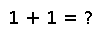# selectperm

selectperm(v, k, [alg=,] [by=,] [lt=,] [rev=false])

Return a partial permutation of the the vector `v`, according to the order specified by `by`, `lt` and `rev`, so that `v[output]` returns the first `k` (or range of adjacent values if `k` is a range) values of a fully sorted version of `v`. If `k` is a single index (Integer), an array of the first `k` indices is returned; if `k` is a range, an array of those indices is returned. Note that the handling of integer values for `k` is different from `select` in that it returns a vector of `k` elements instead of just the `k` th element. Also note that this is equivalent to, but more efficient than, calling `sortperm(...)[k]`

## Examples

Checking you are not a robot: